Roman numerals +

Add up the number writtens in Roman numerals. Write the results as a decimal number.

Result

MMCCCLXXXIX + MCDXXXIX =  3828
MCMXCV + MCCLXXXVIII =  3283
CMXXVI + MMDCXCIV =  3620
MDXVII + MDIII =  3020
CCXLIX + MMCCL =  2499
CCII + MMCCCLXXII =  2574
DCCXCVIII + MMCXLI =  2939
CDXX + MMCCLXIX =  2689
CMLXVII + MDCCCLXI =  2828
MDXLIII + MMCDLIV =  3997

Solution:

MMCCCLXXXIX + MCDXXXIX = 2389 + 1439 = 3828
MCMXCV + MCCLXXXVIII = 1995 + 1288 = 3283
CMXXVI + MMDCXCIV = 926 + 2694 = 3620
MDXVII + MDIII = 1517 + 1503 = 3020
CCXLIX + MMCCL = 249 + 2250 = 2499
CCII + MMCCCLXXII = 202 + 2372 = 2574
DCCXCVIII + MMCXLI = 798 + 2141 = 2939
CDXX + MMCCLXIX = 420 + 2269 = 2689
CMLXVII + MDCCCLXI = 967 + 1861 = 2828
MDXLIII + MMCDLIV = 1543 + 2454 = 3997

Leave us a comment of example and its solution (i.e. if it is still somewhat unclear...):Be the first to comment!Next similar examples:

1. Roman numerals 2+Add up the number writtens in Roman numerals. Write the results as a roman numbers.
2. Multiples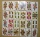What is the sum of the multiples of number 7 that are greater than 30 but less than 56?
3. ProductResult of the product of the numbers 1, 2, 3, 1, 2, 0 is:
4. SummandOne of the summands is 145. The second is 10 more. Determine the sum of the summands.
5. Operations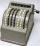Sum of the numbers 1.01 and 3.35 multiply by the difference of numbers 6.69 and 1.39.
6. To thousands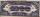Round to thousands following numbers:
7. Decimal expansion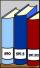Calculate: 2 . 1 + 0 . 10 + 7 . 10000 + 4 . 1000 + 6 . 100 + 0 . 100000 =
8. CollectionMajka gave from her collection of calendars Hanke 15 calendars, Julke 6 calendars and Petke 10 calendars. Still remains 77 calendars. How many calendars had Majka in her collection at the beginning?
9. Tulips and daffodils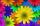Farm cultivated tulips and 211 units on 50 units more daffodils. How many spring flowers grown together?
10. Trees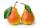In the orchard, 38 apple trees and 20 pear trees grew. How many trees there grow?
11. Shoes 4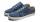Belinda's shoe is 12.45 centimeters long. Felix's shoe is 2.8 centimeters longer than Belinda's shoe. In centimeters, what is the combined length of their two shoes?
12. Roses and tulipsAt the florist are 50 tulips and 5 times less roses. How many flowers are in flower shop?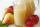Mam used 9 red, 9 green apples and 3 pears to prepare juice. How many pieces of fruit used by mom to prepare this juice?Combine like terms 4c+c-7cAt a weather centre, the temperature at midnight was -2 degree Celsius and by noon it had raised 4 degree Celsius. What is the new temperature?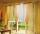For room equipment bought Hanak 50 m curtains. On the dining room needed 90 m and 90 m to hallways of curtains. How many meters of curtains bought totally?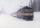The train passes part of the line for 95 minutes at speed 75 km/h. What speed would have to go in order to shorten the driving time of 20 minutes?